## Magnitudes

FAINT                                                                                                                                                                                            BRIGHT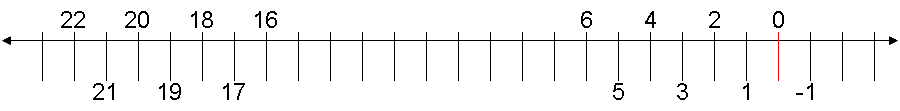The magnitude scale measures the brightness both of stars and of the sky.   The apparent magnitude (m) of visible stars ranges approximately from m=0 for the brighter stars to m=6 for the fainter naked eye stars.  Stars of m=7 and beyond are visible only through a telescope.   A few brilliant stars and celestial objects are actually less than m=0  (that is, negative numbers).  For example, the star Sirius is m= -1.4; the full moon's apparent brightness is about m= -12.5; and the sun is m= -26.

The difference in brightness between each magnitude is a factor of about 2.5.  A star (say, m=4) that is one magnitude brighter than another star (m=5) is 2.5 times brighter.  Hence, a fourth magnitude star is 2.5 times as bright as a fifth magnitude star.  A star five magnitudes greater than another (five times, so 2.5 x 2.5 x 2.5 x 2.5 x 2.5) is 100 times brighter, so a bright first magnitude (m=1) star is 100 times brighter than a faint sixth magnitude (m=6) star.

Stars of m=16 down to m=22 are extremely  faint, requiring powerful telescopes to be seen.  The faintest objects detected by the Hubble Space Telescope are around m=29.  Though out of reach of small telescopes, this range of stellar magnitudes (m=16 to m=22) is used as a guide for measuring the background sky brightness with a Sky Quality Meter (SQM).  Remember, the smaller the magnitude number the brighter the star, as exaggerated below.

Simulated Star Brightness
(in magnitudes)

FAINTER                                                                                                                                                                                  BRIGHTER

 * m=22 * m=21 * m=20 * m=19 * m=18 * m=17 * m=16

To gauge the brightness of the background sky, think about how bright the sky would be if a small area were about as bright as a faint star.

In particular, imagine you were to view a tiny box of sky that measured only 1 second of arc across by 1 second of arc down (or a square arc-second) in area.  A "square arc-second" is sometimes also called an "arc-second squared."  Either way, that's a tiny area.  Consider this: From horizon to horizon is 180 degrees of arc.  Your fist held at arm's length covers about ten degrees of sky, so you could stack about nine fists from the horizon to a point overhead (90 degrees).  One degree of arc is subdivided into 60 minutes of arc, and each minute of arc is subdivided into another 60 seconds of arc.

For comparison, the full moon is half a degree across, or about 30 minutes of arc.  One arc-second is 1/60th of an arc-minute.  So the moon is about 30 arc-minutes x 60 arc-seconds/minute=1800 arc-seconds across.  Again, to measure the sky brightness you want an area only 1 square arc-second, a box whose sides are a tiny fraction (1/1800th) of the moon's diameter.

 * m=21
Now spread the faint light of a star of m=21 (left) across the entire square field of view (the squares at right are exaggerated in size).  The sky behind the stars (right) is slightly brighter than pitch black.  If that background sky brightness were about equal to the smeared light of a 21st magnitude star, you would be looking at nearly the darkest natural skies found on earth.  The Sky Quality Meter (SQM) would read 21.00  magnitudes/square arc-second (or 21 magnitudes/arcsecond2).
 * * * * * * * * *
21
mag/arc-sec2

Unfortunately, the average background sky is brighter than one 21st star per square arc-second.  Remember, as the magnitude number gets smaller, the brightness is greater.  A star of m=16 is 100 times brighter than our example star at m=21.

 * m=16
Similarly, spread the light of a star of m=16 across that entire square field of view.  If the background sky brightness were about equal to the smeared light of a 16th magnitude star (still faint, but significantly brighter than m=21), you would likely be looking at a light polluted sky.  The Sky Quality Meter (SQM) would read 16.00 magnitudes/square arc-second (or 16 magnitudes/arcsecond2).  .
 * * * * * * * * *
16
mag/arc-sec2

Measuring Sky Brightness with an SQM
(
in magnitudes per square arc-second, or "squims")

 * * * * * * * * *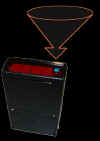22

mag/arc-sec2

 * * * * * * * * *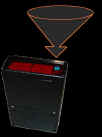21

mag/arc-sec2

 * * * * * * * * *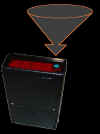20 mag/arc-sec2

 * * * * * * * * *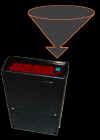19 mag/arc-sec2

 * * * * * * * * *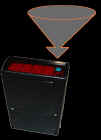18 mag/arc-sec2

 * * * * * * * * *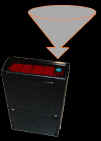17 mag/arc-sec2

 * * * * * * * * *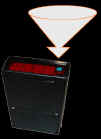16 mag/arc-sec2

 (FAINTER)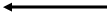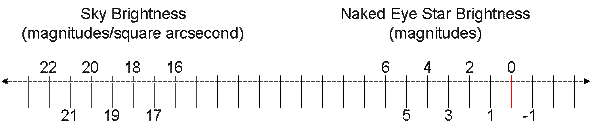(BRIGHTER)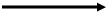21 mag/arc-sec2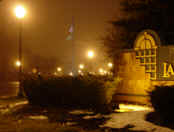16 mag/arc-sec2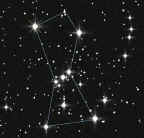m= 1m= -26

If you have read this far, you have done well, for the concept of "magnitudes per square arc-second" is not easy to visualize.  For the purpose of Night Vision, we will make up a simpler name for the Sky Quality Meter (SQM) units and call them "squims."  For example, if the meter reads 18.75 magnitudes per square arc-second, we will describe it as "18.75 squims."  Any objections?

"The greater the squim, the greater the dim."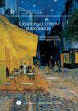"The practical minimum of the natural background radiation is ~21.6 magnitude per square arcsecond," per authors Kohei Narisada and Duco Schreuder.  Source:  Light Pollution Handbook, page 61.

From http://www.astropix.com/HTML/L_STORY/SKYBRITE.HTM:
How Dark Can the Night Sky Get? by Brian Skiff
"The widely accepted value for sky brightness at the zenith at a site completely free of man-made light sources and near solar activity minimum is: V mag. 22.0 per square arcsecond = mag. 13 per square arcminute. In other words, a perfect site has a sky brightness equivalent to having a mag. 22 star in every square arcsecond box (hardly bigger than the star image itself) over the entire sky."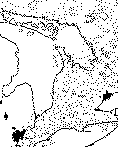http://www.rasc.ca/light/print/berry.htm
Light Pollution in Southern Ontario by Richard Berry includes Tables I and II , which describes the appearance of the sky for given SQM values (in magnitudes per arcsecond squared).

From the Journal of the Royal Astronomical Society of Canada, Vol. 70, No. 3, June 1976, Whole, No. 540; reprinted courtesy of Toronto Centre, R.A.S.C.